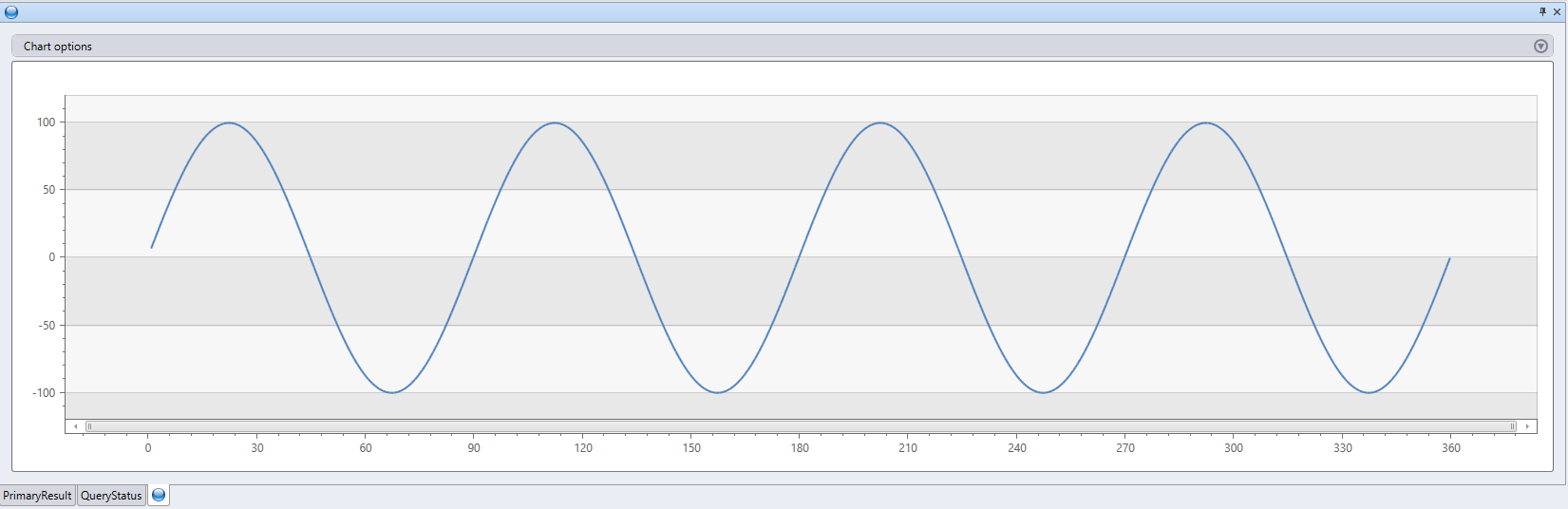# R 插件（预览版）

R 插件使用 R 脚本运行用户定义的函数 (UDF)。 此脚本获取表格数据作为其输入，并生成表格输出。 插件的运行时承载在群集节点上的沙盒中。 沙盒提供隔离的安全环境。

## 语法

T `|` `evaluate` [`hint.distribution` `=` (`single` | `per_node`)] `r(`output_schema`,` script [`,` script_parameters]`)`

## 参数

• output_schema：`type` 文本，定义由 R 代码返回的表格数据的输出架构。
• 格式为：`typeof(`ColumnName`:` ColumnType[, ...]`)`，例如：`typeof(col1:string, col2:long)`
• 若要扩展输入架构，请使用以下语法：`typeof(*, col1:string, col2:long)`
• script`string` 文本，是要执行的有效 R 脚本。
• script_parameters：可选的 `dynamic` 文本，它是一个包含名称/值对的属性包，作为保留的 `kargs` 字典传递给 R 脚本。 有关详细信息，请参阅保留的 R 变量
• hint.distribution：一个可选的提示，用于在多个群集节点上分布插件的执行。 默认：`single`
• `single`：脚本的单个实例将针对整个查询数据运行。
• `per_node`：如果在分发 R 块之前执行查询，则脚本的一个实例将在每个节点上针对其包含的数据运行。

## 保留的 R 变量

• `df`：输入表格数据（上述 `T` 的值），采用 R 数据帧形式。
• `kargs`：script_parameters 参数的值，采用 R 字典形式。
• `result`：R 脚本创建的 R 数据帧。 此值将成为发送到插件后面的任何 Kusto 查询运算符的表格数据。

## 说明和限制

• R 沙盒映像基于 R 3.4.4 for Windows，并且包括来自 Anaconda's R Essentials 捆绑包的程序包。
• R 沙盒限制对网络的访问。 R 代码无法动态安装映像中未包含的其他程序包。 如果你需要特定的程序包，请在 Azure 门户中 新建支持请求

## 示例

``````range x from 1 to 360 step 1
| evaluate r(
//
typeof(*, fx:double),               //  Output schema: append a new fx column to original table
//
'result <- df\n'                    //  The R decorated script
'n <- nrow(df)\n'
'g <- kargs\$gain\n'
'f <- kargs\$cycles\n'
'result\$fx <- g * sin(df\$x / n * 2 * pi * f)'
//
, pack('gain', 100, 'cycles', 4)    //  dictionary of parameters
)
| render linechart
``````## 性能提示

• 将插件的输入数据集减少到所需的最小量（列/行）。

• 请尽可能通过 Kusto 查询语言对源数据集使用筛选器。
• 若要对源列的子集进行计算，请在调用插件之前仅投影这些列。
• 如果脚本中的逻辑可分发，请使用 `hint.distribution = per_node`

• 请尽可能使用 Kusto 查询语言来实现 R 脚本的逻辑。

例如：

``````.show operations
| where StartedOn > ago(1d) // Filtering out irrelevant records before invoking the plugin
| project d_seconds = Duration / 1s // Projecting only a subset of the necessary columns
| evaluate hint.distribution = per_node r( // Using per_node distribution, as the script's logic allows it
typeof(*, d2:double),
'result <- df\n'
'result\$d2 <- df\$d_seconds\n' // Negative example: this logic should have been written using Kusto's query language
)
| summarize avg = avg(d2)
``````

## 使用提示

• 若要避免 Kusto 字符串分隔符与 R 字符串分隔符之间的冲突，请使用以下方法：

• 为 Kusto 查询中的 Kusto 字符串文本使用单引号字符 (`'`)。
• 为 R 脚本中的 R 字符串文本使用双引号字符 (`"`)。
• 使用 externaldata 运算符获取存储在外部位置（例如 Azure Blob 存储或公共 GitHub 存储库）中的脚本内容。

例如：

``````let script =
externaldata(script:string)
[h'https://kustoscriptsamples.blob.core.chinacloudapi.cn/samples/R/sample_script.r']
with(format = raw);
range x from 1 to 360 step 1
| evaluate r(
typeof(*, fx:double),
toscalar(script),
pack('gain', 100, 'cycles', 4))
| render linechart
``````

Azure Monitor 不支持此功能